## Heaviside Step Function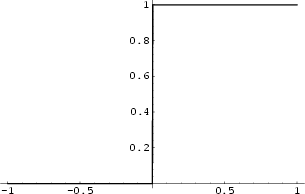A discontinuous step'' function, also called the Unit Step, and defined by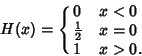(1)

It is related to the Boxcar Function. The Derivative is given by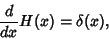(2)

where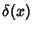is the Delta Function, and the step function is related to the Ramp Function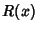by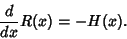(3)

Bracewell (1965) gives many identities, some of which include the following. Letting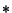denote the Convolution,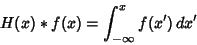(4)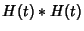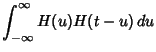(5)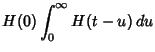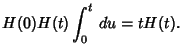(6)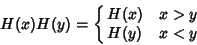(7)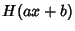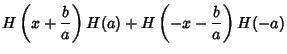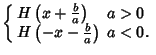(8)

The step function obeys the integral identities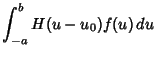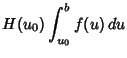(9)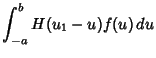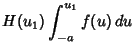(10)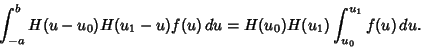(11)

The Heaviside step function can be defined by the following limits,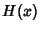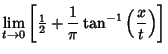(12)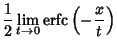(13)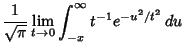(14)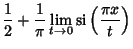(15)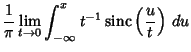(16)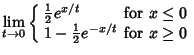(17)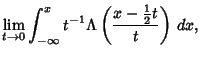(18)

where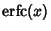is the Erfc function,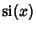is the Sine Integral,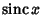is the Sinc Function, and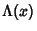is the one-argument Triangle Function and

The Fourier Transform of the Heaviside step function is given by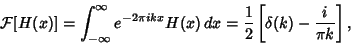(19)

where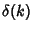is the Delta Function.

See also Boxcar Function, Delta Function, Fourier Transform--Heaviside Step Function, Ramp Function, Ramp Function, Rectangle Function, Square Wave

References

Bracewell, R. The Fourier Transform and Its Applications. New York: McGraw-Hill, 1965.

Spanier, J. and Oldham, K. B. The Unit-Step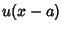and Related Functions.'' Ch. 8 in An Atlas of Functions. Washington, DC: Hemisphere, pp. 63-69, 1987.